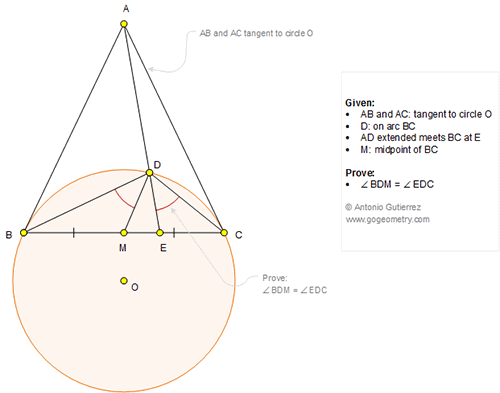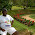## Thursday, October 6, 2016

### Geometry Problem 1273 Circle, Tangent, Secant, Midpoint, Isogonal Lines, Congruent Angles

Geometry Problem. Post your solution in the comment box below.
Level: Mathematics Education, High School, Honors Geometry, College.

Click the figure below to view more details of problem 1273.1.https://goo.gl/photos/bYZ9Rdpg2VBXLXcB8

Using pole and polar properties:
Let DE and DM cut circle O at F and G,
Let MA cut circle O at H
We have OM. OA= OC^2=OD^2
So A is the pole of BC w.r.t circle O
And A and E are harmonic conjugate points of D and F or ( A,E, D,F)= -1
Since ∠EMA= 90 so Apollonius circle diameter AE will pass through M
So ME is a angle bisector of angle MDF.
And ∠AMD=∠FMH=∠HMG => BC//GF
So Arc BG=Arc CF and ∠BDM=∠EDC

2.https://goo.gl/photos/bYZ9Rdpg2VBXLXcB8

Using elementary geometry:
Let DE and DM cut circle O at F and G,
Let MA cut circle O at H
We have OM. OA= OC^2=OD^2
So OA/OF=OF/OM => triangle MOF similar to triangle FOA ( case SAS)
And FA/FO=FM/MO….. (1)
Similarly triangle MOD similar to triangle DOA ( case SAS)
And DA/DO=MD/MO ….. (2)
Divide (1) to (2) and note that OD=OF
We have FA/DA=FM/MD => MA is a external angle bisector of angle DMF
And ∠AMD=∠FMH=∠HMG => BC//GF
So Arc BG= Arc CF and angle BDM= angle CDE

3.Problem 1273
If AD and DM cut circle O at K and L.Then AB^2=AM.AO=AC^2=AD.AK. So MOKD is cyclic.
OD^2=OB^2=OM.OA so triangle ADO is similar with triangle DMO.Therefore <OMD=<ODA
or <AMD=<ODK=<OMK. So <KMC=<CMD =<KMD/2=<KOD/2=<KBD, then <CMD=<CBD+<BDL=<KMC=<CBD+<CBK. So <BDL=<KBC=<KDC.
APOSTOLIS MANOLOUDIS 4 HIGH SCHOOL KORYDALLOS PIRAEUS GREECE

4.Reading thro the 2nd proof of Peter, give below a slightly modified approach

Let AD meet the circle at P.
Let < MAP = p, < PAC = q, < ACD = < DBC = < DPC = r and < ODP = < OPD = s

Then OC^2 = OM. OA = OD^2 = OP^2
So < MDO = < MPA = p hence OMDP is concyclic

Therefore < AMD = s and so
< BDM = 90-s-r .....(1)

Now < DOC = 2r so < CDE = 90-r-s (since < ODP = s)

Therefore < BDM = < CDE = 90-s-r

Sumith Peiris
Moratuwa
Sri Lanka

5.How about the converse "if < BDM = < CDE then M is the mid point"?

6.The symmedian passes through the intersection of the tangents to the circumcircle at the extremities of the opposite side, i.e. AD is symmedian of tr. DBC.

7.Extend AD to meet the circle O at X.Connect AMO and form the right triangle ABO
In the triangle ABO
AB^2 = AM.AO
But AB^2 = AD.AX
Hence D,M,O and X are concyclic points.

Let Angle XDC = EDC = @ and ODX = \$
=> Angle OXD = \$ => OMD = 180-\$ => AMD = \$ => BMD = 90+\$ -------------(1)
Join OC and consider the isosceles triangle ODC
We know Angle ODC = @+\$ = OCD => Angle DOC = 180-2(@+\$) => Angle DBC = DBM = DOC/2 = 90-(@+\$)----------(2)

Now consider the triangle BDM and from (1) and (2)
Angle BDM = 180-(90+\$)-(90-(@+\$)) = @ = EDC

8.Let DM extended meet the circle at X and let ADE extended meet the circle at Y. Let AMO extended meet XY at Z.

OM.OA = OB^2 = OD^2 = OY^2

So < OAY = < MDO = < MYO hence OMDY is concyclic with OD = OY and so OM bisects < XMY.

Easily MOZ is perpendicular to XY and so BC//XY

Therefore BX = CY in isosceles trapezoid BCYX and the result follows

Sumith Peiris
Moratuwa
Sri Lanka